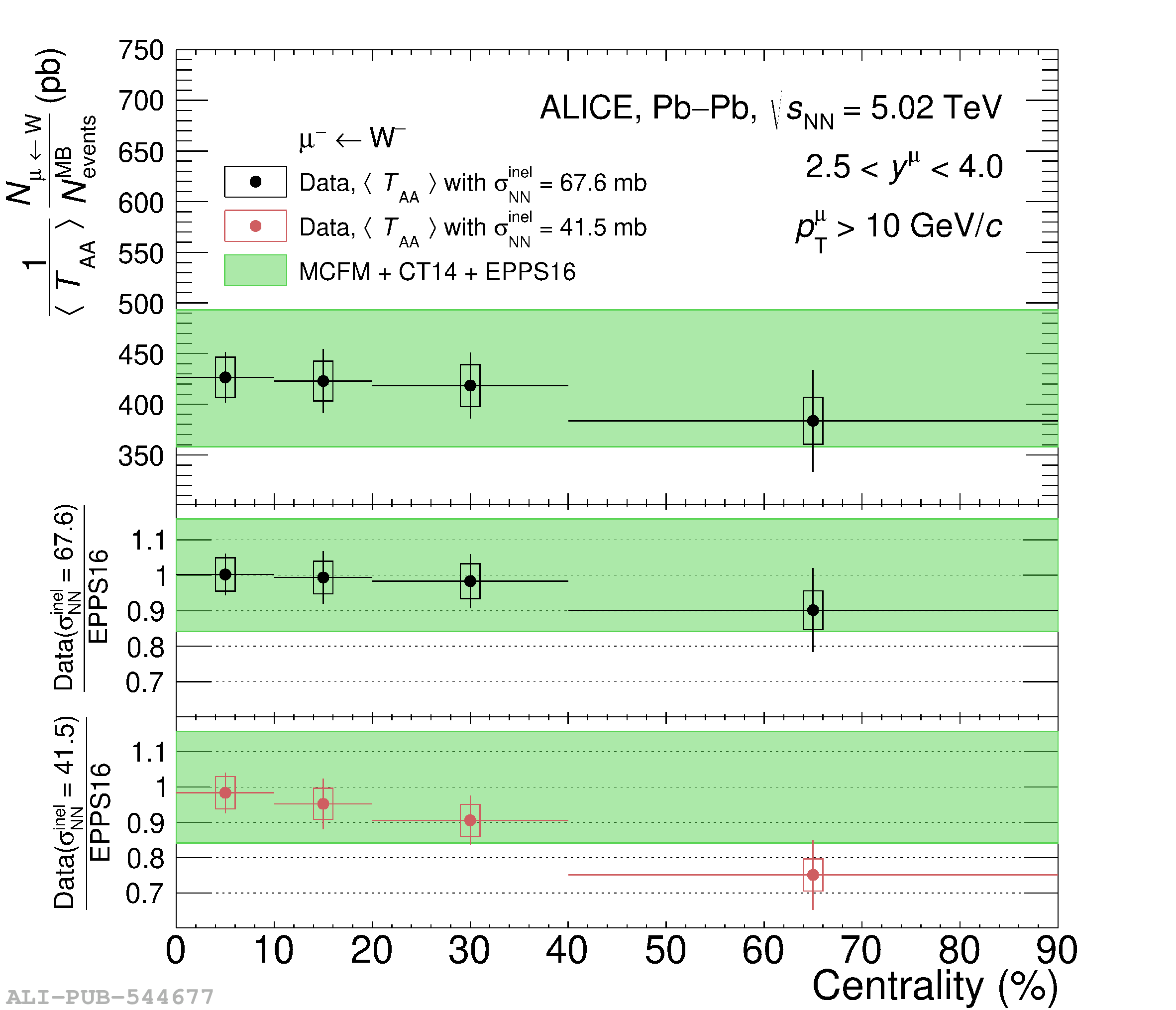# Figure 9

 \avTaa-scaled yield of muons from \Wminus (left) and \Wplus (right) decays in \PbPb collisions at \fivenn for muons with $\pt^\mu > 10$ \GeVc and $2.5 < y^\mu_{\rm cms} < 4.0$. In the top panels the yield is compared with pQCD calculations using the CT14 PDF  as baseline and implementing the EPPS16  nuclear modifications. The ratio to theory of the measured yield normalised with \avTaa evaluated with $\sigma^{\rm inel}_{\rm NN}$ = 67.6 mb and 47.5 mb is shown in the middle and bottom panels, respectively (see the text for details). The horizontal bars indicate the width of the centrality intervals, the vertical bars and boxes correspond to the statistical and systematic uncertainties, respectively. The band indicate the uncertainty on the theoretical computations.